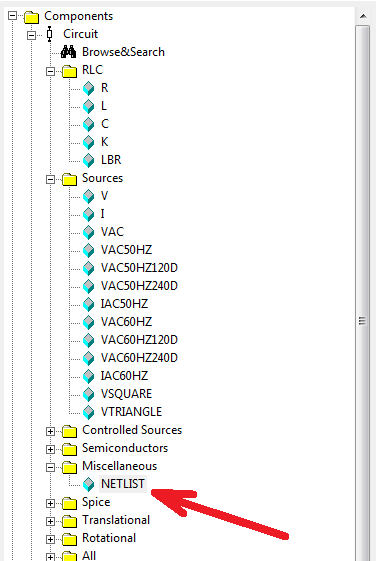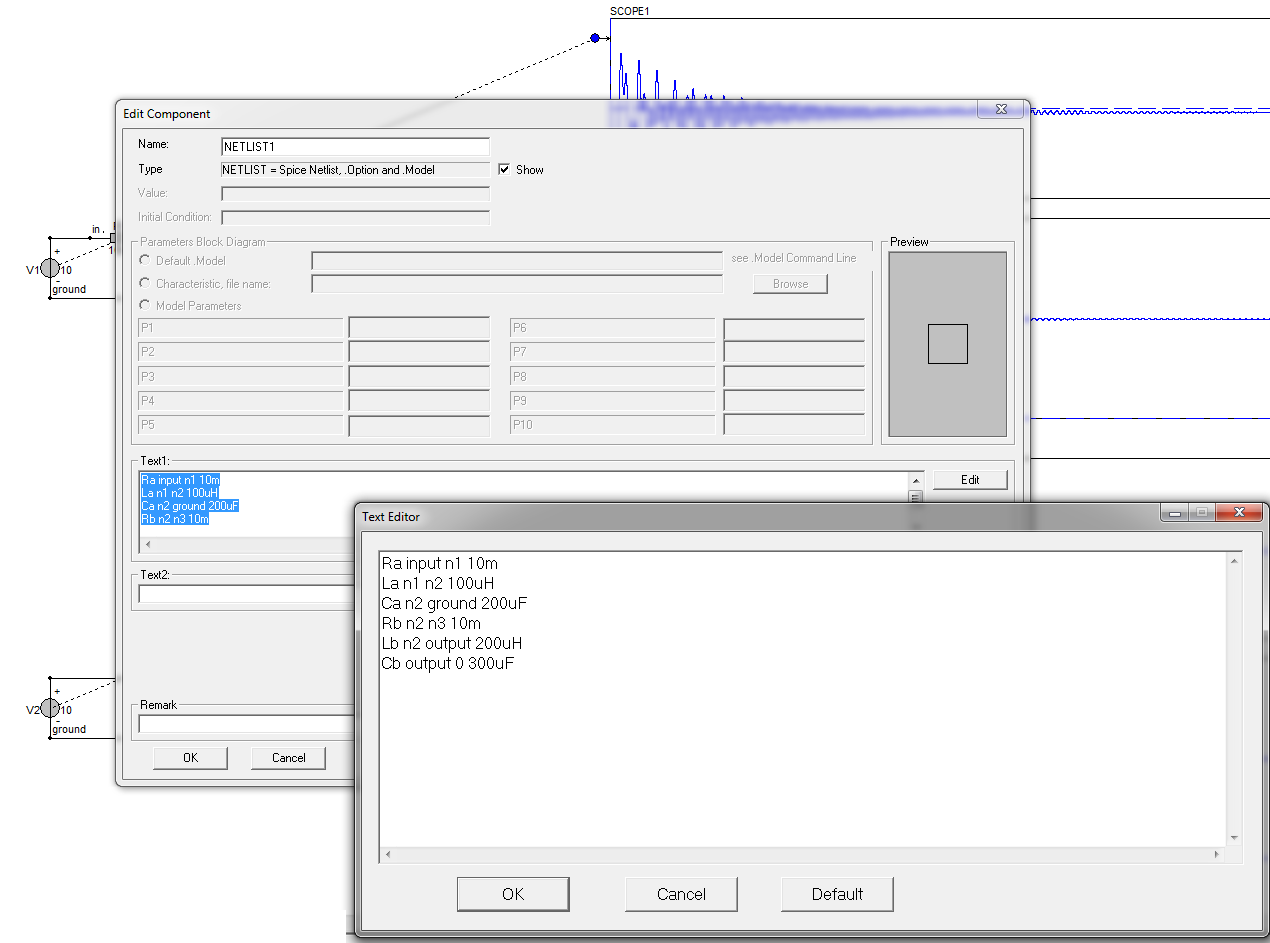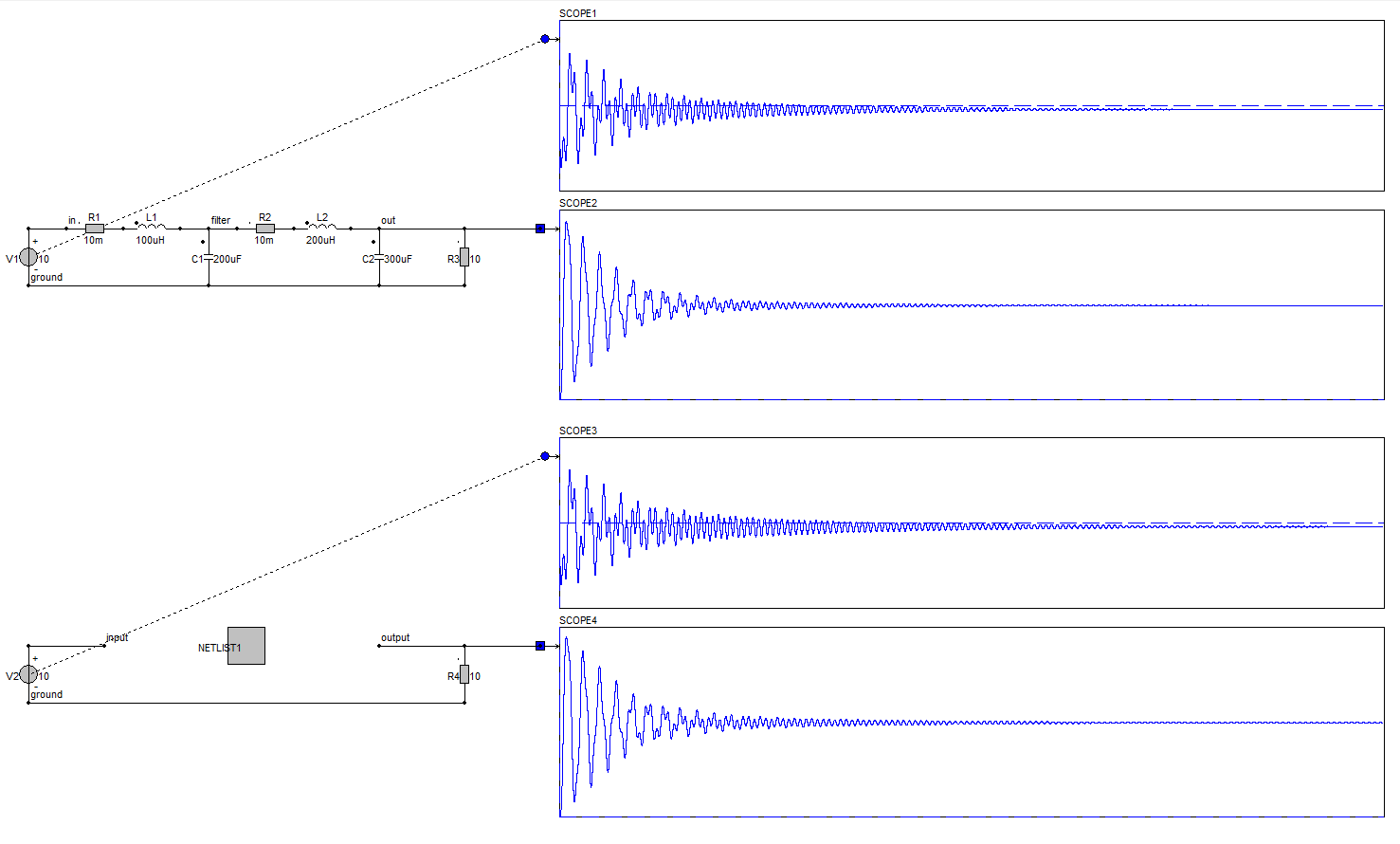• Introduction
• What is in this manual
• What is Caspoc
• User interface
• Introduction
• Starting
• Simulation
• Editing
• Viewing and printing
• Getting Started
• Basic editing
• Simulation in the time domain
• Basic User Interface Topics
• Editing
• Simulation
• Viewing
• Library
• Reports
• Project management
• Circuit and Block Diagram Components
• Introduction
• Cscript and user defined functions
• Component parameters
• Modeling Topics
• Introduction
• Power Electronics
• Semiconductors
• Electrical Machines
• Electrical drives
• Power Systems
• Mechanical Systems
• Thermal Systems
• Magnetic Circuits
• Green Energy
• Coupling to FEM
• Experimenter
• Analog hardware description language
• Embedded C code Export
• Coupling to Spice
• Small Signal Analysis
• Matlab coupling
• Tips and tricks
• Appendices

## Import a Spice netlist into Caspoc.

In this section we will import a Spice netlist into Caspoc. We will demonstrate this by first modeling the complete circuit using standard Caspoc models in the schematic editor and comparing this to an imported netlist.

The schematic contains two LC filters with different resonant frequencies. Damping is introduced by specifying the series resistance of both inductors.We can rebuild this circuit by selecting the Netlist component from the circuit sectionWe enter the same circuit, but now as a spice netlist. Notice that all labels and nodes must be different fro the ones that we used in the schematic editor, except when we want to connect nodes.finally we can compare the simulation results and we can see that they are equal.